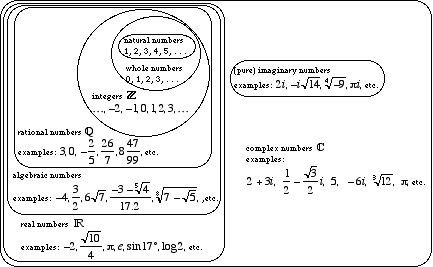index: click on a letter A B C D E F G H I J K L M N O P Q R S T U V W X Y Z A to Z index index: subject areas numbers & symbols sets, logic, proofs geometry algebra trigonometry advanced algebra & pre-calculus calculus advanced topics probability & statistics real world applications multimedia entrieswww.mathwords.com about mathwords website feedback

 Algebraic Numbers Real numbers that can occur as roots of polynomial equations that have integer coefficients. For example, all rational numbers are algebraic. So are all surds such as $$\sqrt 7$$, as well as numbers built from surds such as the number below. $\frac{{42 + \sqrt{{15.2}}}}{{\sqrt {4 - \sqrt 3 } }}$. Note: Real numbers which are not algebraic are known as transcendental numbers.See also